# Practice Questions with Solutions: Introduction to Numbers Notes | Study Mathematics for Class 4 - Class 4

## Class 4: Practice Questions with Solutions: Introduction to Numbers Notes | Study Mathematics for Class 4 - Class 4

The document Practice Questions with Solutions: Introduction to Numbers Notes | Study Mathematics for Class 4 - Class 4 is a part of the Class 4 Course Mathematics for Class 4.
All you need of Class 4 at this link: Class 4

Question 1: Write the number name for each of the following numbers :
(a) 38,946
(b) 2,90,534
(c) 4,59,861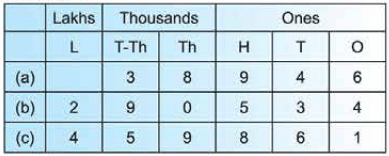• Thirty-eight thousand, nine hundred forty six.
• Two lakh, ninety thousand, five hundred thirty-four.
• Four lakh, fifty nine thousand, eight hundred sixty-one

Note: The plural for the period is not written. For example, instead of writing six lakhs, forty thousands, two hundreds, we write six lakh, forty thousand, two hundred.

Question 2: Find the face-value of:
(a) 2 in 1,95,263
(b) 5 in 25,134
(c) 8 in 1,63,548
(d) 6 in 60,053

(a) Face-value of 2 in 1,95,263 is 2.

(b) Face-value of 5 in 25,134 is 5.

(c) Face-value of 8 in 1,63,548 is 8.

(d) Face-value of 6 in 60,053 is 6.

Question 3: Which is greater?
(a) 2,537 or 51,693
(b) 5,12,964 or 291
(c) 1,56,201 or 27,193

(a) 2,537 has 4 digits and 51 ,693 has 5 digits.
So, 51,693 is greater than 2,537 or 51,693 > 2,537.
(b) 5,12,964 has 6 digits and 291 has 3 digits.
So, 5,12,964 is greater than 291 or 5,12,964 > 291.
(c) 1, 56,201 has 6 digits and 27,193 has 5 digits.
So, 1,56,201 is greater than 27,193 or 1,56,201 > 27,193.

Question 4: Write the number- 'two lakh, five thousand, one hundred twenty-four' in figures.

We proceed as follows:
Step 1: Make three periods as shown.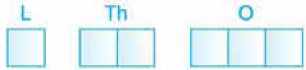The first period from right will have three parts, the second will have two parts and the third will have only one part.

Step 2: Write all the lakhs in the first period from the left.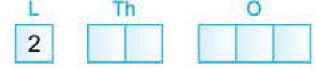Step 3: Write all the thousands in the second period from the left. We fill all the parts. For example, if it is 5 thousand, we write 05.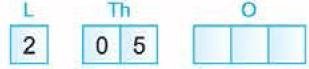Step 4: Write the hundreds, tens and ones in the third period from the left.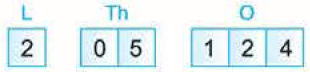Question 5: Write the place-value of 3 in each of the following numbers :
(a) 13,584 (b) 3,50,962 (c) 6,85,931 (d) 5,93,821

Arrange the digits of the numbers in the place-value chart.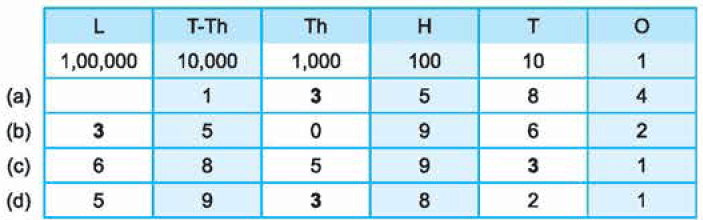(a) Place-value of 3 in 13,584= 3 x 1,000 = 3,000.
(b) Place-value of 3 in 3,50,962= 3 x 1,00,000 = 3,00,000.
(c) Place-value of 3 in 6,85,931 = 3 x 10 = 30.
(d) Place-value of 3 in 5,93,821 = 3 x 1,000 = 3,000.

Question 6: Compare :
(a) 25,306 and 25,419
(b) 6,34,510 and 6,34,578

(a) Consider 25,306 and 25,419. Both are 5-digit numbers.
We start from the left-most digits. Here, 2 = 2.
Next, we compare the second digits from the left. Here, 5 = 5.
Next, we compare the third digits from the left. Here, 3 < 4
Thus, 25,306 < 25,419 or 25,419 > 25,306.
(b) Consider 6,34,510 and 6,34,578. Both are 6-digit numbers.
Comparing the digits, we have
6 = 6 (left-most digits)
3 = 3 (second digits from the left)
4 = 4 (third digits from the left)
5 = 5 (fourth digits from the left)
1 < 7 (fifth digits from the left)
Thus, 6,34,510 < 6,34,578 or 6,34,578 > 6,34,510.

Question 7: Write each of the following numbers in figures :
(a) Twelve thousand, five hundred twenty-one.
(b) Forty-one thousand, two.
(c) Six lakh, thirty-six thousand, five hundred ten.
(d) Eight lakh, three thousand, fifty-six.

(a) Twelve thousand, five hundred twenty-one.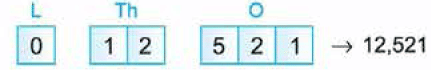(b) Forty-one thousand, two.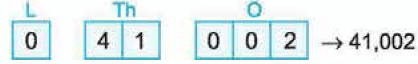(c) Six lakh thirty-six thousand, five hundred ten.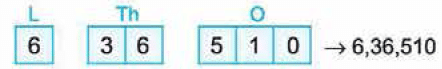(d) Eight lakh, three thousand, fifty-six.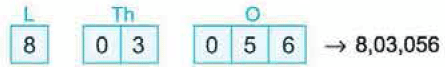Question 8 : Write the place-value of 0 in the following numbers :
(a) 25,170
(b) 6,52,701
(c) 3,05,217

(a) In 25,170, 0 is at ones place, so its place-value is 0 x 1 = 0.
(b) In 6,52, 701, 0 is at tens place, so its place-value is 0 x 10  = 0.
(c) In 3,05,217, 0 is at ten thousands place, so its place-value is 0 x 10,000 = 0.

Question 9: Arrange the following numbers in ascending order :
3,25,102; 5,65,101; 4,32,256; 7,41,212; 4,63,205

First we arrange the given numbers in a place value chart :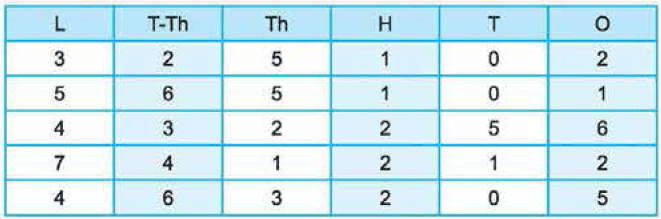All the numbers have 6 digits. Comparing the digits at the lakhs place, we get 3 < 4 < 5 < 7.
Now we compare 4,32,256 and 4,63,205. Both these numbers have the same digits at the lakhs place. So, we compare the digits at the ten-thousands place.
This digit is 3 in 4,32,256 and 6 in 4,63,205.
Since, 3 < 6, so, 4,32,256 < 4,63,205.
Thus, 3,25,102 < 4,32,256 < 4,63,205 < 5,65,101 < 7,41,212
Hence, the given numbers arranged in ascending order are :
3,25,102; 4,32,256; 4,63,205; 5,65,101; 7,41,212.

Descending order means the numbers from the greatest to the smallest.

Question 10: Write the following numbers in expanded form : (a) 35,126 (b) 3,01,647 Solution :

Write the given numbers in the place-value chart.
(a)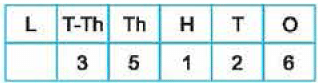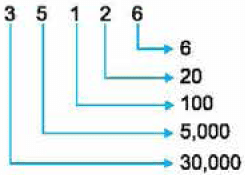Thus, 35,126 = 30,000 + 5,000 + 100 + 20 + 6.
(b)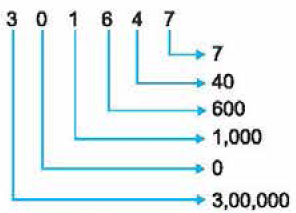Thus, 3,01,647 =3 ,00,000 + 0 + 1,000 + 600 + 40 + 7
=3 ,00,000 + 1,000 + 600 + 40 + 7.

Question 11: Arrange the following numbers in descending order :
4,69,901; 90,618; 6,06,211; 5,58,690; 6,26,923

First we arrange the given numbers in a place value chart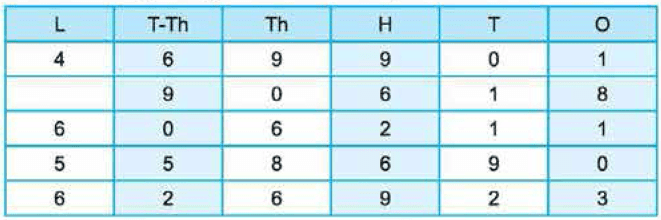90,618 is a 5-digit number. All others are 6-digit numbers.
So, 90,618 is the smallest number.
Comparing the digits at lakhs place, we get 6 > 5 > 4.
Now, we compare 6,06,211 and 6,26,923. Both the numbers have 6 at the lakhs place. So, we compare their digits at the ten-thousands place.
This digit is 0 in 6,06,211 and 2 in 6,26,923.
So, 6,06,211 < 6,26,923.
Thus, 6,26,923 > 6,06,211 > 5,58,690 > 4,69,901 > 90,618.
Hence, the given numbers arranged in descending order are:
6,26,923; 6,06,211; 5,58,690; 4,69,901; 90,618.

Question 12: Find the successors of:
(a) 599
(b) 3,500
(c) 31,499

(a) Successor of 599 = 599 + 1 = 600.
(b) Successor of 3,500 = 3,500 + 1 = 3,501.
(c) Successor of 31,499 = 31,499 + 1 = 31,500.

Question 13: Write the smallest and the greatest 4-digit numbers using all the digits 3, 6 and 5. You may repeat the digits.

The smallest 4-digit number = 3,356
Note that here we repeated the smallest digit.
The greatest 4-digit number= 6,653
Note that here we repeated the greatest digit.

Question 14: Find the predecessor of :
(a) 270
(b) 6,900
(c) 7,54,196

(a) Predecessor of 270 = 270- 1 = 269.
(b) Predecessor of 6,900 = 6,900 - 1 = 6,899.
(c) Predecessor of 7,54,196 = 7,54,196- 1 = 7,54,195.

Question 15: Write the smallest and the greatest 4-digit number using two different digits.

Using two smallest digits are 0 and 1.
Then, the smallest 4-digit number is 10 00.
Using two greatest digits are 9 and 8.
Then, the greatest 4-digit number= 9998.

The document Practice Questions with Solutions: Introduction to Numbers Notes | Study Mathematics for Class 4 - Class 4 is a part of the Class 4 Course Mathematics for Class 4.
All you need of Class 4 at this link: Class 4Use Code STAYHOME200 and get INR 200 additional OFF

## Mathematics for Class 4

15 videos|68 docs|32 tests

Track your progress, build streaks, highlight & save important lessons and more!

,

,

,

,

,

,

,

,

,

,

,

,

,

,

,

,

,

,

,

,

,

;